#### Nondeterministic forward projections

To facilitate the notation, suppose in this section that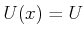for all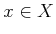. In Section 10.1.3 this will be lifted.

Suppose that the initial state,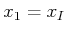, is known. If the action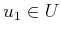is applied, then the set of possible next states is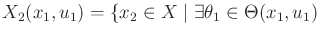such that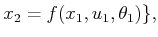(10.10)

which is just a special version of (10.5). Now suppose that an action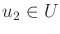will be applied. The forward projection must determine which states could be reached from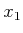by applying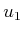followed by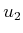. This can be expressed as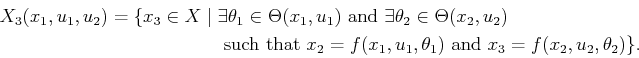(10.11)

This idea can be repeated for any number of iterations but becomes quite cumbersome in the current notation. It is helpful to formulate the forward projection recursively. Suppose that an action history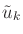is fixed. Let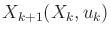denote the forward projection at stage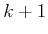, given that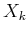is the forward projection at stage. This can be computed as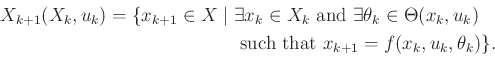(10.12)

This may be applied any number of times to compute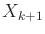from an initial condition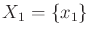.

Example 10..3 (Nondeterministic Forward Projections)   Recall the first model given in Example 10.1, in which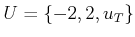and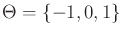. Suppose that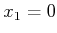, and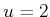is applied. The one-stage forward projection is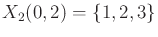. Ifis applied again, the two-stage forward projection is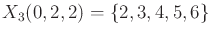. Repeating this process, the-stage forward projection is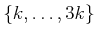.Steven M LaValle 2020-08-14